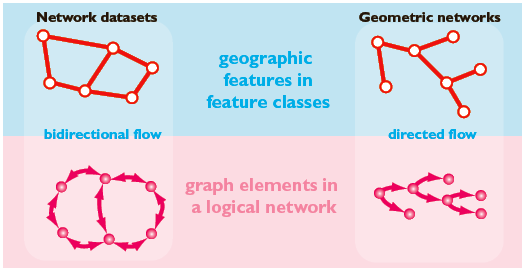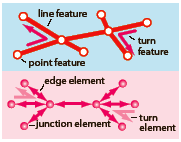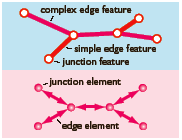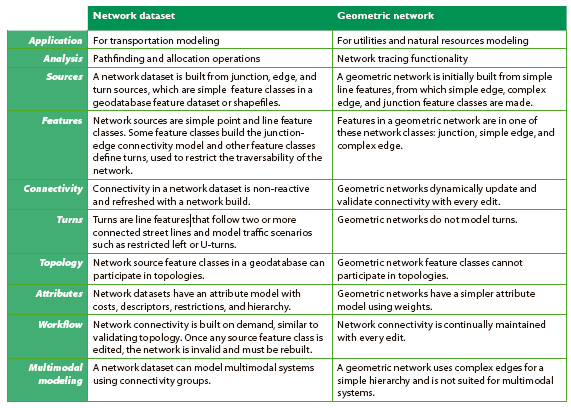﻿ Network datasets and geometric networks—ArcObjects 10.4 Help for Java | ArcGIS for Desktop

# Network datasets and geometric networks

Summary
Networks model the transportation of people and resources, such as water, electricity, gas, and communications. Networks constrain flow to edges, such as streets and river reaches, which join at junctions, such as intersections and confluences. The geodatabase has two core network models—the network dataset models transportation networks and the geometric network models directed-flow systems—such as, river networks and utility lines.

### Models that represent networks

The geodatabase implements two core models for representing networks—geometric networks and network datasets. Geometric networks are designed for directed networks, such as an electric utility or a river network. Network datasets are optimized for undirected networks, especially transportation networks.
Network datasets and geometric networks contain geographic features in feature classes from which a graph is derived. The elements of this graph are stored in a logical network and a set of element and connectivity tables. There are differences in how features are defined in the two models and how operational workflows refresh the graph, but both involve the same conceptual abstraction of a graph from geographic features. See the following illustration:### Network datasets

Network datasets are more analogous to a geodatabase topology—a network dataset has network sources defined on simple features. These features undergo no modification and can also participate in a topology. With network datasets, you can update connectivity on demand with a rebuild of the entire network.
Network datasets better model undirected networks. They allow flow in any direction and employ turns to model restrictions.
Network datasets have an attribute environment that uses costs, descriptors, restrictions, and hierarchy. Network datasets are optimized for large transportation networks. Network datasets are made from simple line, point, and turn features. Connectivity properties fine-tune how elements are discovered from features. See the following illustration:Transportation networks in ArcGIS are modeled as network datasets. Analysis of transportation networks, such as shortest-path discovery, is modeled by ArcGIS Network Analyst extension. The network dataset application programming interface (API) is in the network dataset section of the Geodatabase library.

### Geometric networks

Geometric networks use custom network features (simple edge, complex edge, and junction) to model the parts of a network. Complex edges are used to model a compound set of edges and junctions, such as an electrical distribution line with connected services.
With geometric networks, connectivity is updated whenever you add or remove any network feature. When you edit features in a geometric network, the logical network is continuously updated.
Geometric networks have connectivity rules that control which network objects can properly connect to each other. Analytical solvers use weights (impedances on edges) to perform tracing functions useful to utility and hydrographic applications. Solvers include downstream trace, upstream trace, isolation trace, and path trace.
The following illustration shows a simple geometric network with one complex edge, and two simple edges are represented with five edge elements:Utility and river networks in ArcGIS are modeled as geometric networks. The geometric network API is in the geometric network section of the Geodatabase library.

### Key differences between network datasets and geometric networks

A key difference between geometric networks and network datasets is how network connectivity is maintained.
• A geometric network is made initially from simple line and point features, which become junction, simple edge, and complex edge features. Once created, network connectivity is continuously updated during edits.
• A network dataset has network sources from which a connectivity model is discovered on demand.
Geometric networks and network datasets have logical networks. A logical network is a set of tables representing network elements and their connectivity. For geometric networks, the logical network is incrementally updated through edits. For network datasets, you regenerate the logical network with every network build.
This following illustration summarizes the qualities of the two network models: